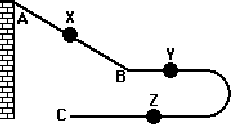# Acceleration Read from Lesson 1 of the 1-d kinematics chapter at The Physics Classroom

 Date 19.05.2018 Size 34.59 Kb.

Motion in One Dimension Name:

Acceleration
Read from Lesson 1 of the 1-D Kinematics chapter at The Physics Classroom:
http://www.physicsclassroom.com/Class/1DKin/U1L1e.cfm
MOP Connection: Kinematic Concepts: sublevels 4 and 7
Review:

The instantaneous velocity of an object is the speed of the object with a direction

The Concept of Acceleration

1. Accelerating objects are objects that are changing their velocity. Name the three controls on an automobile that cause it to accelerate.

Acceleration is a change in velocity - either in the speed or in the direction. The brake pedal and gas pedal cause speed changes. The steering wheel causes direction changes.
2. An object is accelerating if it is moving _____. Circle all that apply.

a. with changing speed b. extremely fast c. with constant velocity

d. in a circle e. downward f. none of these
Accelerating objects are changing their velocity. A velocity is a speed with a direction. An object with a changing velocity can be changing its speed (choice a) and/or changing its direction (choice d).
3. If an object is NOT accelerating, then one knows for sure that it is ______.

a. at rest b. moving with a constant speed

c. slowing down d. maintaining a constant velocity
If an object is not accelerating, then it is not changing its velocity. The velocity is constant. A velocity is a speed with a direction; so if it has a constant velocity, then it also has a constant speed and direction.
Acceleration as a Rate Quantity

Acceleration is the rate at which an object's velocity changes. The velocity of an object refers to how fast it moves and in what direction. The acceleration of an object refers to how fast an object changes its speed or its direction. Objects with a high acceleration are rapidly changing their speed or their direction. As a rate quantity, acceleration is expressed by the equation:

acceleration = =
4. An object with an acceleration of 10 m/s2 will ____. Circle all that apply.

a. move 10 meters in 1 second b. change its velocity by 10 m/s in 1 s

c. move 100 meters in 10 seconds d. have a velocity of 100 m/s after 10 s
5. Ima Speedin puts the pedal to the metal and increases her speed as follows: 0 mi/hr at 0 seconds; 10 mi/hr at 1 second; 20 mi/hr at 2 seconds; 30 mi/hr at 3 seconds; and 40 mi/hr at 4 seconds. What is the acceleration of Ima's car?
Ima's acceleration is 10 mi/hr/s because her speed changes by 10 mi/hr each second.
6. Mr. Henderson's (imaginary) Porsche accelerates from 0 to 60 mi/hr in 4 seconds. Its acceleration is 15 mi/hr/s.

a. 60 mi/hr b. 15 m/s/s c. 15 mi/hr/s d. -15 mi/hr/s e. none of these

7. A car speeds up from rest to +16 m/s in 4 s. Calculate the acceleration.
a = ∆velocity / time = (16 m/s - 0 m/s)/(4 s) = (16 m/s) / (4 s) = 4 m/s2
8. A car slows down from +32 m/s to +8 m/s in 4 s. Calculate the acceleration.
a = ∆velocity / time = (8 m/s - 32 m/s)/(4 s) = (-24 m/s) / (4 s) = - 6 m/s2

Acceleration as a Vector Quantity

Acceleration, like velocity, is a vector quantity. To fully describe the acceleration of an object, one must describe the direction of the acceleration vector. A general rule of thumb is that if an object is moving in a straight line and slowing down, then the direction of the acceleration is opposite the direction the object is moving. If the object is speeding up, the acceleration direction is the same as the direction of motion.

9. Read the following statements and indicate the direction (up, down, east, west, north or south) of the acceleration vector.

 Description of Motion Dir'n of Acceleration a. A car is moving eastward along Lake Avenue and increasing its speed from 25 mph to 45 mph. Eastward b. A northbound car skids to a stop to avoid a reckless driver. Southward c. An Olympic diver slows down after splashing into the water. Upward d. A southward-bound free quick delivered by the opposing team is slowed down and stopped by the goalie. Northward e. A downward falling parachutist pulls the chord and rapidly slows down. Upward f. A rightward-moving Hot Wheels car slows to a stop. Leftward g. A falling bungee-jumper slows down as she nears the concrete sidewalk below. Upward

 10. The diagram at the right portrays a Hot Wheels track designed for a phun physics lab. The car starts at point A, descends the hill (continually speeding up from A to B); after a short straight section of track, the car rounds the curve and finishes its run at point C. The car continuously slows down from point B to point C. Use this information to complete the following table.Point Direction of Velocity of Vector Direction of Acceleration Vector X Rightward Reason: The velocity is always in the direction of motion. Rightward Reason: If an object speeds up, its accel'n is in the direction of motion. Y Rightward Reason: The velocity is always in the direction of motion. Leftward Reason: If an object slows down, then its accel'n is opposite its motion. Z Leftward Reason: The velocity is always in the direction of motion. Rightward Reason: If an object slows down, then its accel'n is opposite its motion.

© The Physics Classroom, 2009 Page